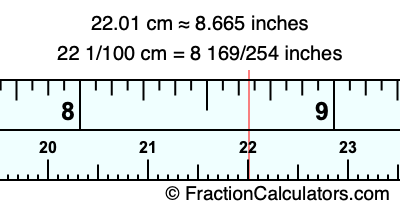22.01 cm in inchesHere is how to convert 22.01 cm to inches. We will give you the fractional answer, the decimal answer, and illustrate the answer on our tape measure.

One centimeter is equal to 50/127 inches, and 22.01 as a fraction is 22 1/100. Therefore, to get the fractional answer to 22.01 cm in inches, we multiply 22 1/100 by 50/127 and simplify if necessary.

cm × 50/127 = inches
22 1/100 × 50/127 = inches
22 1/100 × 50/127 = 8 169/254
22 1/100 cm = 8 169/254 inches
22.01 cm = 8 169/254 inches

As promised above, we also have the answer to 22.01 cm in inches in decimal form. Although the fractional answer above is exact, the decimal answer may be rounded if necessary:

8 169/254 ≈ 8.66535433070866
8 169/254 ≈ 8.665
22.01 cm ≈ 8.665 inches

Our image below shows 22.01 cm on a tape measure. The top row of the tape measure is inches, and the bottom row is centimeters.As you can see, we drew a red line where 22.01 cm and 8.665 inches meet on the measuring tape.

Centimeters to Inches Calculator
Here you can convert another length in centimeters to inches.

Convert  cm to inches.

What is 22.02 cm in inches?
Here is another inches to centimeters calculation we did for you.